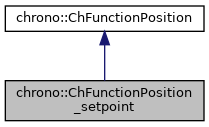chrono::ChFunctionPosition_setpoint Class Reference

## Description

A motion function p=f(s) where p(t) is an externally-provided sample, as a ZOH (zero order hold) of FOH (first order).

You must keep the setpoint p updated via multiple calls to SetSetpoint(), for example calling SetSetpoint() at each timestep in the simulation loop. It is assumed that one will later evaluate Get_p(), Get_p_ds() etc. in close vicinity of the setpoint (old setpoints are not saved), preferably ecactly at the same s, but in vicinity of s there will be extrapolation (after last s) and interpolation (before last s) according to: If in ZOH mode: value p will persist indefinitely until next call, derivative p_ds and p_dsds will be zero. If in FOH mode: value p will interpolate linearly from the previous value, derivative p_ds will be constant, p_dsds will be zero. If in SOH mode: value p will interpolate quadratically, derivative p_ds will be linear, p_dsds will be constant. Default: uses FOH mode. Use SetMode() to change it.

#include <ChFunctionPosition_setpoint.h>

Inheritance diagram for chrono::ChFunctionPosition_setpoint:[legend]
Collaboration diagram for chrono::ChFunctionPosition_setpoint:[legend]

## Public Types

enum  eChSetpointMode { ZOH, FOH, SOH, OVERRIDE }
Type of setpoint interpolation/extrapolation - zero order hold, first order hold, etc. More...

## Public Member Functions

ChFunctionPosition_setpoint (const ChFunctionPosition_setpoint &other)

virtual ChFunctionPosition_setpointClone () const override
"Virtual" copy constructor.

void SetMode (eChSetpointMode mmode)
Sets the extrapolation/interpolation mode.

eChSetpointMode GetMode ()
Gets the extrapolation/interpolation mode.

void Reset (double ms=0)
Use this to go back to s=0 (the SetSetpoint() function works only if called at increasing s values)

virtual void SetSetpoint (ChVector<> p_setpoint, double s)
Set the setpoint, and compute its derivatives (speed, acceleration) automatically by backward differentiation (only if s is called at increasing small steps, most often s is time). More...

virtual void SetSetpointAndDerivatives (ChVector<> p_setpoint, ChVector<> p_setpoint_ds, ChVector<> p_setpoint_dsds)
Set the setpoint, and also its derivatives. More...

virtual ChVector Get_p (double s) const override
Return the p value of the function, at s, as p=f(s).

virtual ChVector Get_p_ds (double s) const override
Return the dp/ds derivative of the function, at s.

virtual ChVector Get_p_dsds (double s) const override
Return the ddp/dsds double derivative of the function, at s.

virtual void ArchiveOUT (ChArchiveOut &marchive) override
Method to allow serialization of transient data to archives.

virtual void ArchiveIN (ChArchiveIn &marchive) override
Method to allow de-serialization of transient data from archives.Public Member Functions inherited from chrono::ChFunctionPosition
ChFunctionPosition (const ChFunctionPosition &other)

virtual void Estimate_s_domain (double &smin, double &smax) const
Return an estimate of the domain of the function argument. More...

virtual void Estimate_boundingbox (ChVector<> &pmin, ChVector<> &pmax) const
Return an estimate of the range of the function value. More...

virtual void Update (const double t)
Update could be implemented by children classes, ex. to launch callbacks.

## ◆ eChSetpointMode

Type of setpoint interpolation/extrapolation - zero order hold, first order hold, etc.

Enumerator
ZOH

Zero Order Hold: p constant, p_ds = p_dsds = 0 in neighbour of setpoint s.

FOH

First Order Hold: p linear, p_ds constant, p_dsds = 0 in neighbour of setpoint s.

SOH

First Order Hold: p parabolic, p_ds linear, p_dsds constant in neighbour of setpoint s.

OVERRIDE

p, p_ds, p_dsds are set via SetSetpointAndDerivatives() and will be considered constant in Get_p(s) regardless of s until next SetSetpointAndDerivatives()

## ◆ SetSetpoint()

 void chrono::ChFunctionPosition_setpoint::SetSetpoint ( ChVector<> p_setpoint, double s )
virtual

Set the setpoint, and compute its derivatives (speed, acceleration) automatically by backward differentiation (only if s is called at increasing small steps, most often s is time).

Note: each time must be called with increasing s so that internally it add sthe 'new' setpoint and scrolls the previous samples for computing extrapolation/interpolation, but if called multiple times with exactly the same s value, the buffer of past samples is not scrolled: it just recompute setpoint and derivatives according to the 'updated' setpoint. If in ZOH mode: value p will persist indefinitely until next call, derivative p_ds and p_dsds will be zero. If in FOH mode: value p will interpolate linearly from the previous value, derivative p_ds will be constant, p_dsds will be zero. If in SOH mode: value p will interpolate quadratically, derivative p_ds will be linear, p_dsds will be constant.

## ◆ SetSetpointAndDerivatives()

 virtual void chrono::ChFunctionPosition_setpoint::SetSetpointAndDerivatives ( ChVector<> p_setpoint, ChVector<> p_setpoint_ds, ChVector<> p_setpoint_dsds )
inlinevirtual

Set the setpoint, and also its derivatives.

Moreover, changes the mode to eChSetpointMode::OVERRIDE, so all values will persist indefinitely until next call, that is multiple calls to Get_p(s) Get_p_ds() etc. will give same results (non interpolated) regardless of s.

The documentation for this class was generated from the following files:
• /builds/uwsbel/chrono/src/chrono/motion_functions/ChFunctionPosition_setpoint.h
• /builds/uwsbel/chrono/src/chrono/motion_functions/ChFunctionPosition_setpoint.cpp